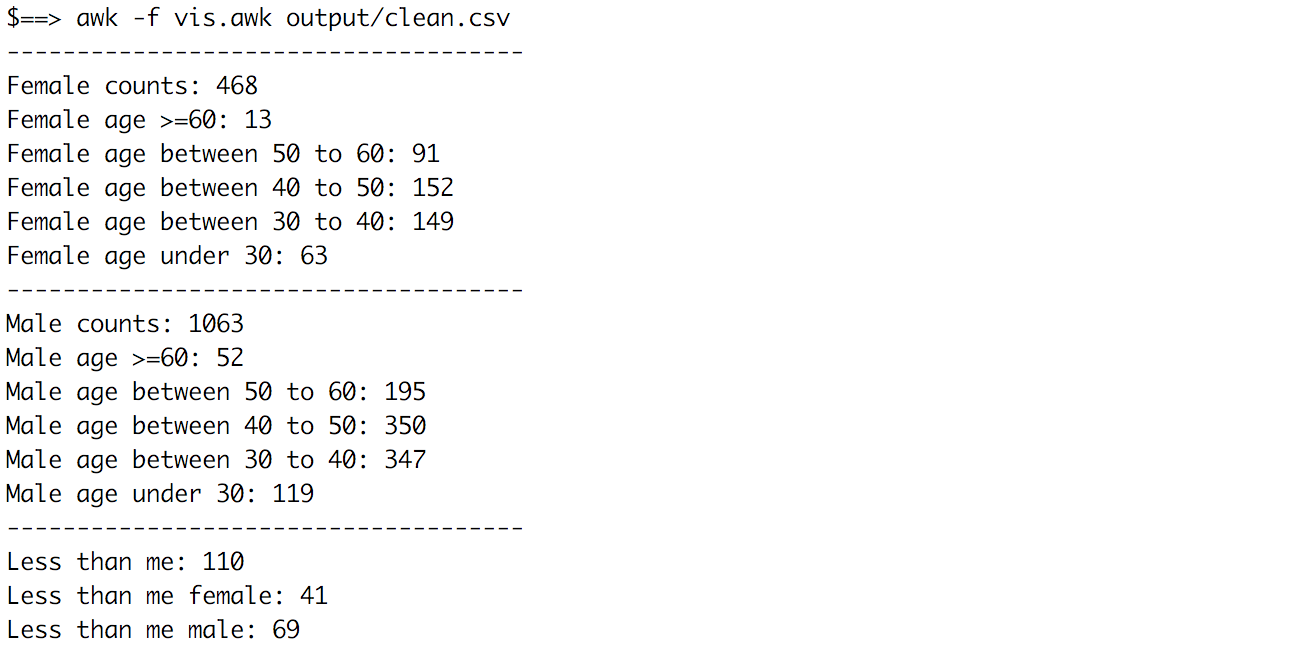Since I will be attending Half Ironman santa cruz 70.3 race, I would love to know something about our atheletes. The online website provides the registered candidates information. So just with a bit hack, you will know something.

``````#!/usr/bin/awk -f
#
# awk -f vis.awk output/clean.csv
#

BEGIN {
FS=","
F_Count=0
F_60_Plus=0
F_50_to_60=0
F_40_to_50=0
F_30_to_40=0
F_30_Minus=0

M_Count=0
M_60_Plus=0
M_50_to_60=0
M_40_to_50=0
M_30_to_40=0
M_30_Minus=0

Less_than_me=0
Less_than_me_M=0
Less_than_me_F=0
}

{
if(\$3 ~ /F/){
# print \$0
F_Count++
if ( \$4 >= 60){
F_60_Plus++
} else if (\$4 >= 50) {
F_50_to_60++
} else if (\$4 >= 40) {
F_40_to_50++
} else if (\$4 >= 30) {
F_30_to_40++
} else if (\$4 < 30) {
F_30_Minus++
}
} else {
M_Count++
if ( \$4 >= 60){
M_60_Plus++
} else if (\$4 >= 50) {
M_50_to_60++
} else if (\$4 >= 40) {
M_40_to_50++
} else if (\$4 >= 30) {
M_30_to_40++
} else if (\$4 < 30) {
M_30_Minus++
}
}

if (\$4 <28) {
Less_than_me++
}
if (\$4<28 && \$3 ~ /F/) {
Less_than_me_F++
}
if (\$4<28 && \$3 ~ /M/) {
#print \$0
Less_than_me_M++
}
}

END {
print "-------------------------------------"
print "Female counts: " F_Count
print "Female age >=60: " F_60_Plus
print "Female age between 50 to 60: " F_50_to_60
print "Female age between 40 to 50: " F_40_to_50
print "Female age between 30 to 40: " F_30_to_40
print "Female age under 30: " F_30_Minus

print "-------------------------------------"
print "Male counts: " M_Count
print "Male age >=60: " M_60_Plus
print "Male age between 50 to 60: " M_50_to_60
print "Male age between 40 to 50: " M_40_to_50
print "Male age between 30 to 40: " M_30_to_40
print "Male age under 30: " M_30_Minus
print "-------------------------------------"
print "Less than me: " Less_than_me
print "Less than me female: " Less_than_me_F
print "Less than me male: " Less_than_me_M
}
``````

The result is…Et voila!!